# Civil Engineering - Strength of Materials

### Exercise :: Strength of Materials - Section 1

16.

In the cantilever truss as shown in below figure, the horizontal component of the reaction at A, is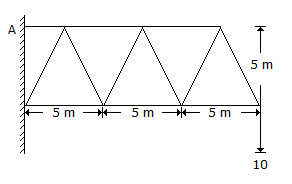A. 30 tonnes B. 60 tonnes C. 90 tonnes D. 120 tonnes E. 150 tonnes.

Explanation:

No answer description available for this question. Let us discuss.

17.

In case of an eccentric loading on a bracket subjected to moment M, the tangential force developed in any rivet, at right angles to its radius vector r is

 A.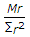B.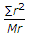C.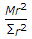D.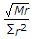Explanation:

No answer description available for this question. Let us discuss.

18.

A simply supported beam of span L carries a concentrated load W at its mid-span. The maximum bending moment M is

 A.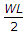B.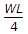C.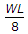D.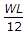E.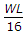Explanation:

No answer description available for this question. Let us discuss.

19.

The ratio of the maximum deflections of a beam simply supported at its ends with an isolated central load and that of with a uniformly distributed load over its entire length, is

 A. 1 B.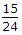C.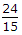D.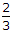E.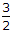Explanation:

No answer description available for this question. Let us discuss.

20.

The shape of the bending moment diagram over the length of a beam, carrying a uniformly distributed load is always

 A. linear B. parabolic C. cubical D. circular.